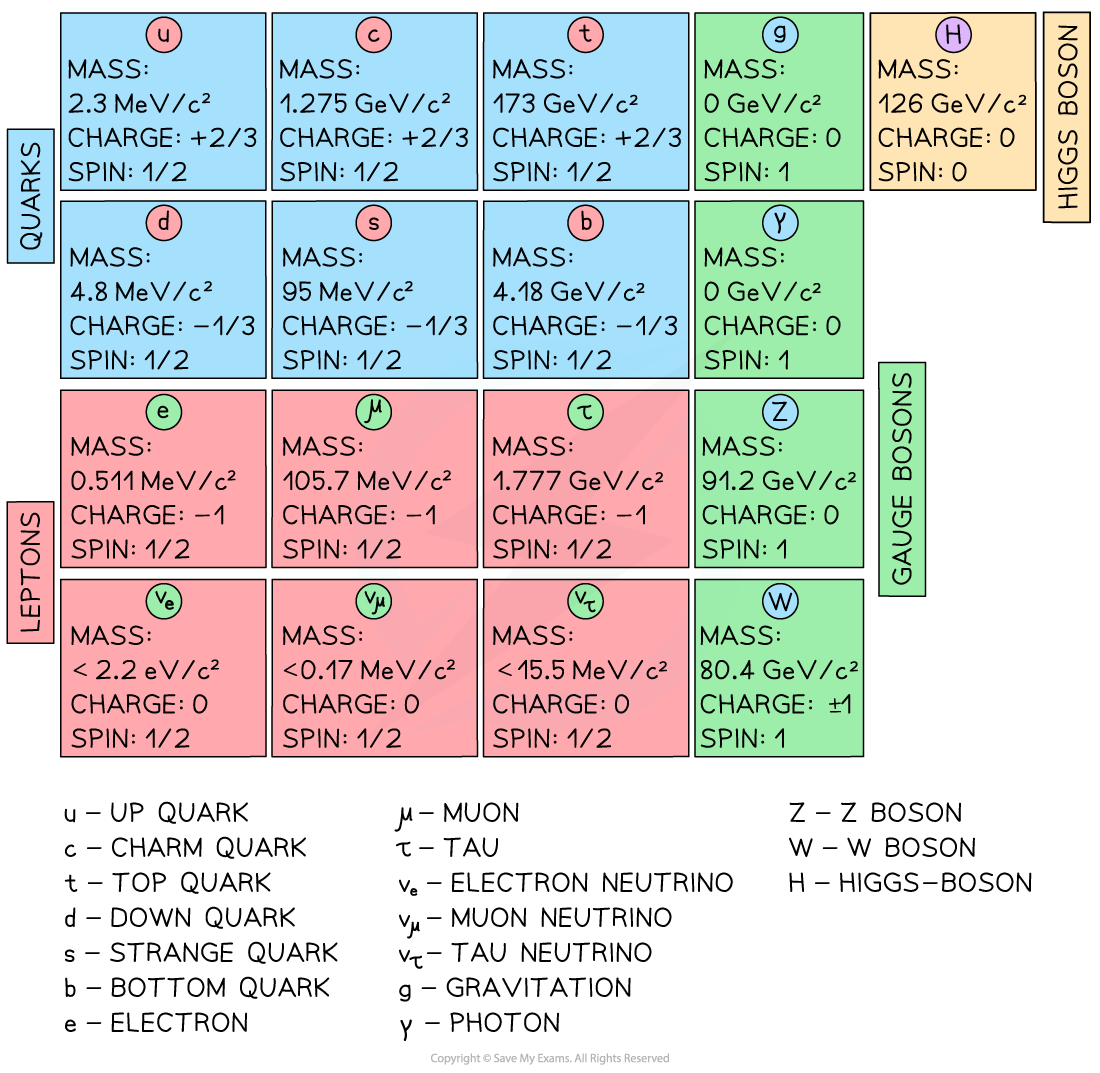# IB DP Physics: HL复习笔记7.3.7 The Standard Model of Particle Physics

### The Higgs Boson

• The Higgs-boson was predicted in 1964
• The prediction included an approximate mass for the particle, at 125 GeV c2
• This prediction came from the standard model, which required the photon, W and Z bosons to be massless
• While the photon is indeed massless, both the W and Z bosons have large masses
• This did not align with the standard model unless mass is not a property of the particle, but a property of space
• The field that gives particles their mass is known as the Higgs-field and the particle associated with this field is the Higgs-boson
• The Higgs-boson was eventually discovered in 2012 at CERN’s Large Hadron Collider (LHC) in Switzerland
• The Higgs-boson is responsible for the mass of all other particles in the standard model
• The quantum field theory that is responsible for this mechanism is beyond the scope of the IB DP Physics course
• Understanding the Higgs-boson mechanism is deeply complex
• However, the concept is an integral part of the standard model and particle physics

### The Standard Model of Particle Physics

• The standard model describes the fundamental particles that make up other sub-atomic particles
• The standard model was developed through experimental discoveries and theoretical predictions
• The latest addition to the standard model was the Higgs-boson which was confirmed in 2012
• Particles are grouped into 4 major categories
• The quarks
• The leptons
• The gauge bosons
• The scalar bosons (or Higgs Boson)
• The particles of the standard model are used to produce larger, non-fundamental particles such as protons and neutronsThe standard model of particle physics

• The standard model explains a lot of the theory of physical interactions in our universe
• Yet, the standard model does not explain
• A complete relativistic theory of gravity
• The existence and nature of dark matter
• The existence and nature of dark energy and the expansion of the universe
• There is still room for improvement on the standard model and scientists continue to seek further answers and refine the current model